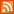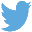Bucaro TecHelpHTTPS Encryption not required because no account numbers orpersonal information is ever requested or accepted by this siteCustom Search# JavaScript Math Object

The JavaScript Math object gives you powerful routines that let you find Square root, logarithms, raise a number to a power, perform trigonometric functions and much more. In addition it provides several properties, such as the value of natural logarithm e, and the value of pi.

Math Object Properties

 Property Value Description Math.E 2.718281828459045091 Natural log Math.LN2 0.6931471805599452862 Natural log of 2 Math.LN10 2.302585092994045901 Natural log of 10 Math.LOG2E 1.442695040888963387 Base-2 log of e Math.LOG10E 0.4342944819032518167 base-10 Log of e Math.PI 3.141592653589793116 pi

Because these properties return their values, you can use them like constants in your mathematical expressions. For example, to obtain the circumference of a circle whose diameter is d, use the statement below:

```circumference = d * Math.PI
```

Math Object Methods

 Method Returns Math.abs(value) Absolute value Math.acos(value) Arc cosine in radians Math.asin(value) Arc sine in radians Math.atan(value) Arc tangent in radians Math.atan2(value1, value2) Angle of polar coordinates x and y Math.ceil(value) Next integer greater than or equal to value Math.cos(value) Cosine Math.exp(value) Raise log e to the power of value Math.floor(value) Next integer less than or equal to value Math.log(value) Natural logarithm (base e) Math.max(value1, value2) The greater of value1 or value2 Math.min(vallue1, value2) The lesser of value1 or value2 Math.pow(vallue1, value2) Value1 to the value2 power Math.random() Random number between 0 and 1 Math.round(value) value + 1 when value >= value.5 otherwise value Math.sin(value) Sine in radians Math.sqrt(value) Square root Math.tan(value) Tangent

Most Math object methods take one or two values as parameters, the only Math object method that doesn't take a value as a parameter is the random method.

 .menubtn { margin-top:10px; margin-left:50px; width:220px; opacity:1; border-style:solid; border-color:#009000; background-color:#66ff66; transition-property: opacity; transition-duration: 1s; transition-timing-function: ease-out; } .menubtn:hover { opacity:1; background-color:#e5ff23; } Menu - More Java Script Quick ReferenceRSS FeedFollow @Stephen Bucaro[Site User Agreement] [Privacy Policy] [Site map] [Search This Site] [Contact Form]
Copyright©2001-2023 Bucaro TecHelp 13771 N Fountain Hills Blvd Suite 114-248 Fountain Hills, AZ 85268